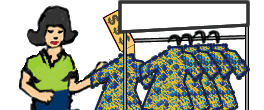# Consumer Math with Percent Applications

## Consumer Math is presented through Percent Applications in this unit.

Lessons include percent and proportions, discount and sale price, simple interest, commission, sales tax and percent increase and decrease. Real-life money problems are used throughout this unit for consumers. Try our sample lessons below, or browse other units.Consumer Math Description Percent and Proportions Students will solve problems of the form "One number is some percent of another number." Discount and Sale Price Students will find the discount and sale price of an item. Real-life consumer problems are used throughout this lesson. Simple Interest Students compute simple interest on a loan or on an investment. Examples illustrate how to compute interest when the time is given in months or in years. Real-life consumer problems are used throughout. Commission Students will compute straight commission as a percentage of sales. Real-life consumer problems are included. Sales Tax Students will compute sales tax as a percentage added to the buyer's cost. Real-life consumer problems are used throughout. Percent Increase and Decrease Students will understand changes in a value over time through percent increase and percent decrease problems. Formulas and examples are presented. Practice Exercises To complete 10 additional exercises as practice with consumer concepts. To assess students' understanding of all consumer problems learned so far. Challenge Exercises To solve 10 additional money problems that challenge students' understanding of consumer concepts. To hone students' problem-solving skills. Solutions To review complete solutions to all exercises presented in this unit. Includes the problem, step-by-step solutions, final answer for each exercise. Learning Objectives Students Will Learn All About Percent And Proportions, Discount And Sale Price, Simple Interest, Commission, Sales Tax, Percent Increase And Decrease.